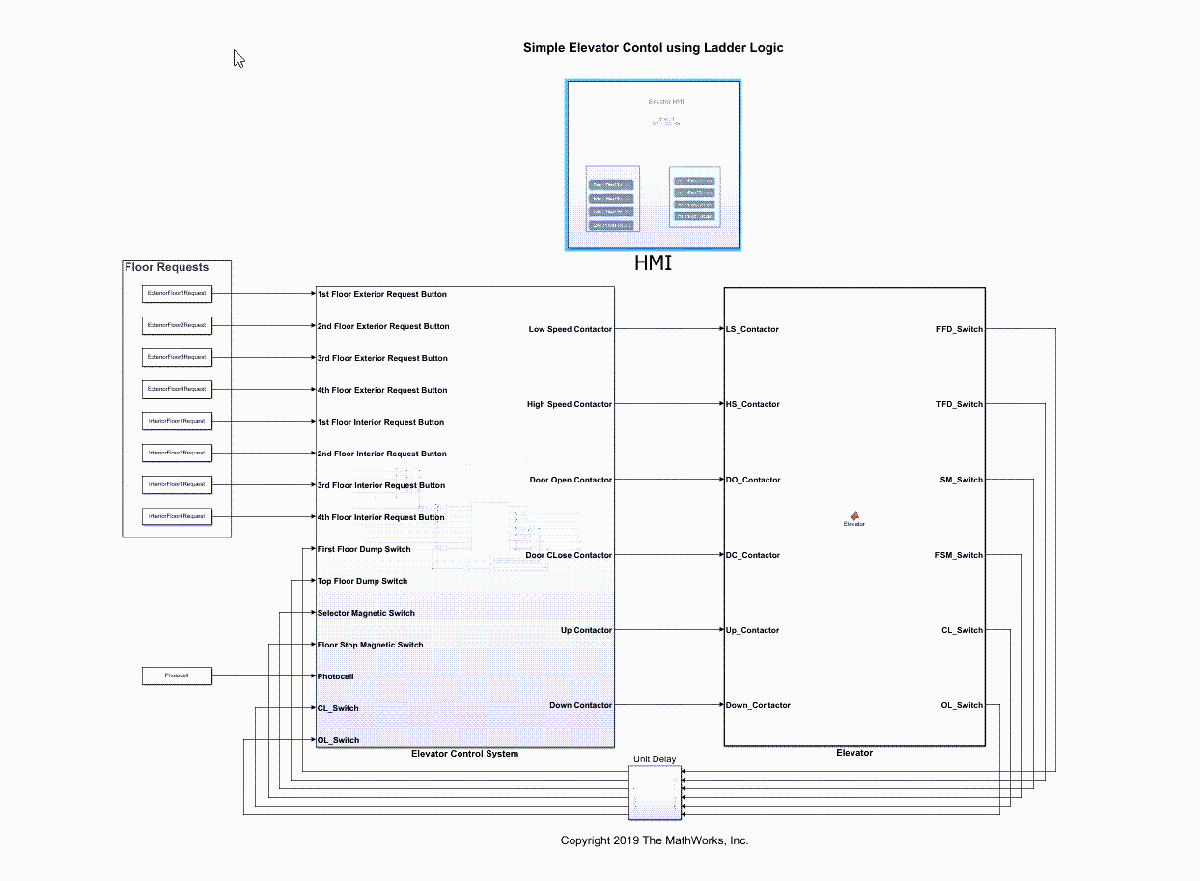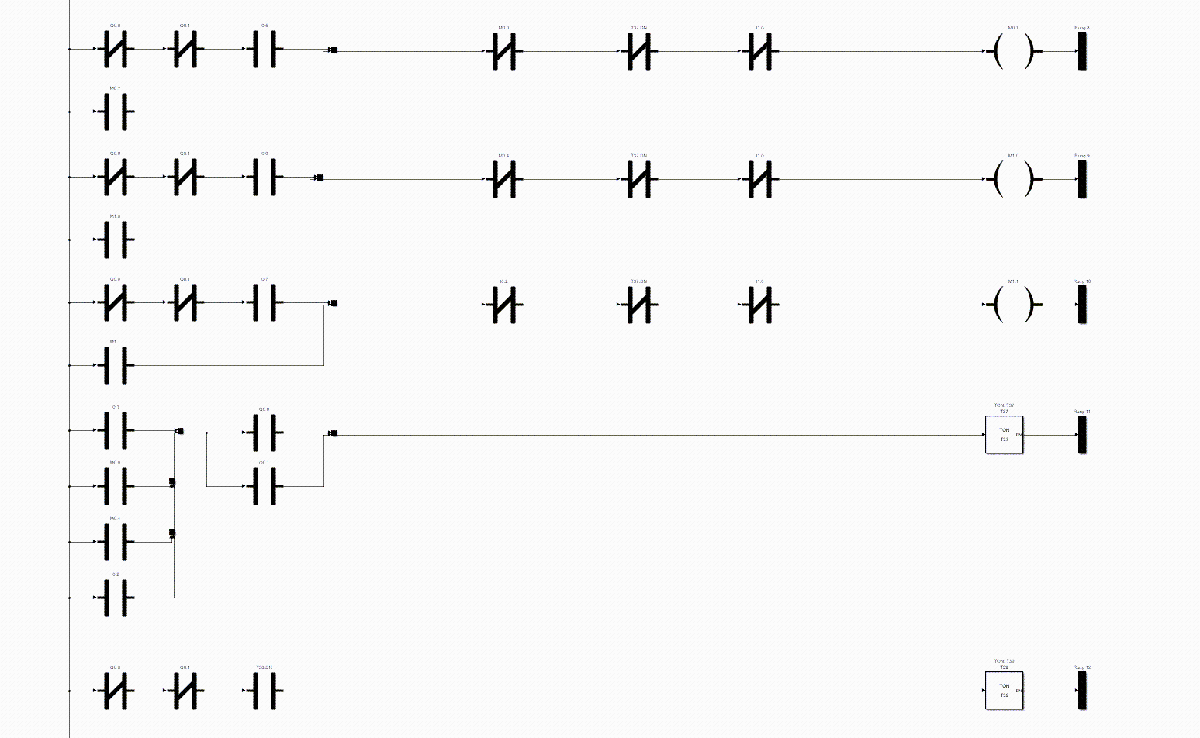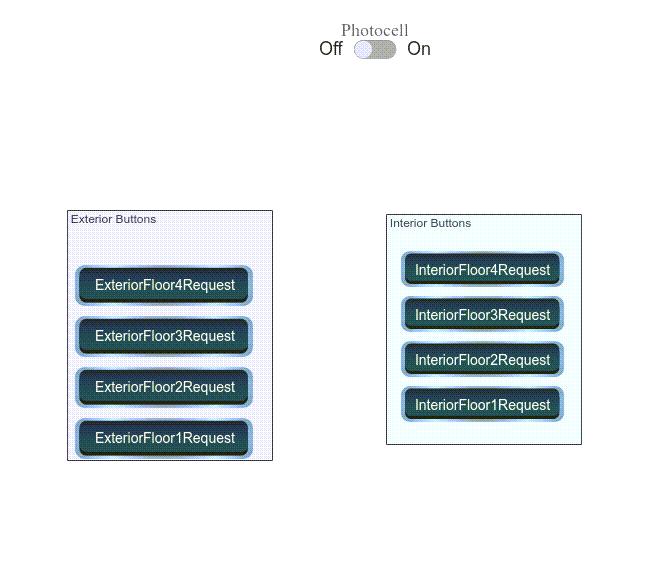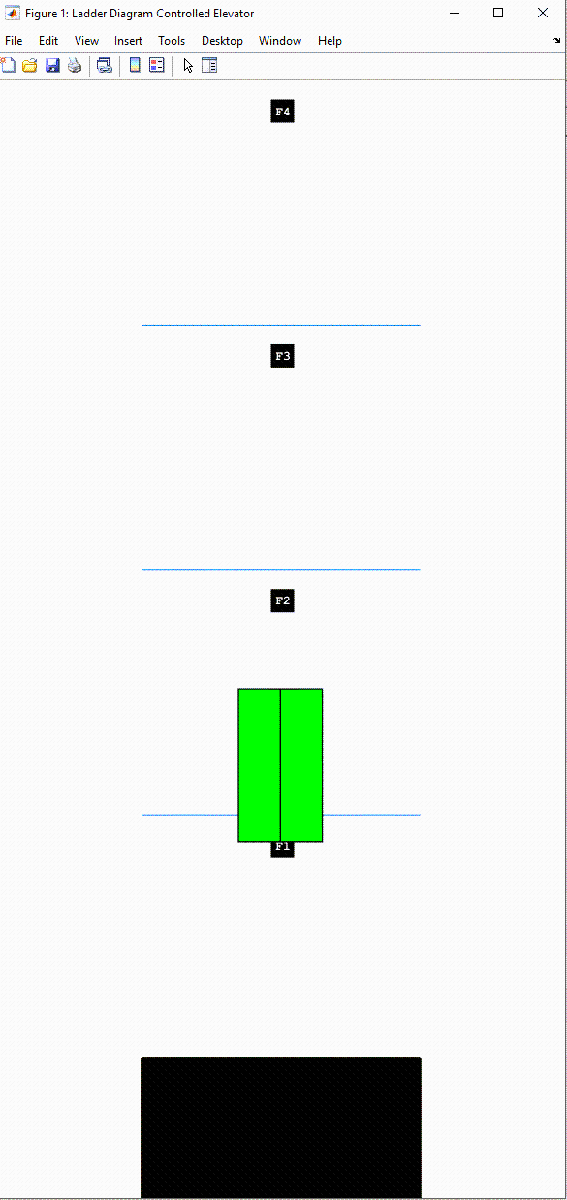# Model, Simulate, and Generate Code for Ladder Logic-Based Elevator Controller

Rapidly prototype the ladder logic-based controller for a single car elevator by using Simulink® PLC Coder™ to model and simulate the ladder logic. After verifying that the controller works for your requirements, generate code for the controller.

### Model Description

The model consists of a `Elevator Control System` block, `Elevator Block`, and a Human Machine Interface (`HMI)` block. Open the model:

`open_system('plcdemo_ladder_elevator');`Opening the model loads the timer configuration, model data types, and initializes the model by using information from the `plcdemo_ladder_elevator_init.m` file.

`type plcdemo_ladder_elevator_init`
```% sample time Ts = 0.1; % load PLC builtin types plcloadtypes; % define timer initial values T37_InitialValue = FB_TON.InitialValue; T37_InitialValue.PRE = int32(300*100); T38_InitialValue = FB_TOF.InitialValue; T38_InitialValue.PRE = int32(100*100); T39_InitialValue = FB_TON.InitialValue; T39_InitialValue.PRE = int32(12*100); T40_InitialValue = FB_TON.InitialValue; T40_InitialValue.PRE = int32(10*100); T41_InitialValue = FB_TOF.InitialValue; T41_InitialValue.PRE = int32(1*100); % define dashboard parameters InteriorFloor1Request = false; InteriorFloor2Request = false; InteriorFloor3Request = false; InteriorFloor4Request = false; ExteriorFloor1Request = false; ExteriorFloor2Request = false; ExteriorFloor3Request = false; ExteriorFloor4Request = false; Photocell = false; ```

#### Elevator Control System Block

The `Elevator Control System` block is a PLC Controller block. It contains a Ladder Program block that contains the ladder logic. To view the ladder logic for the controller, open:

• The` Elevator Control System` block

• The `Elevator PLC Ladder Diagram System` block

• The `Elevator Controller `block

• The `Task` block

• The `Program` block

This image shows a section of the ladder diagram logic inside the `Program` block.#### HMI Block

The `HMI Block` enables you to interact with the model simulation. Open the `HMI` block by double-clicking the block. This image shows the components of the `HMI` block.The `HMI` block contains:

• Exterior Buttons: Represents the exterior floor request buttons.

• Interior Buttons: Represents the interior floor request buttons.

• Photocell: Represents the door sensor.

#### Elevator Block

The `Elevator` block is a MATLAB Function Block that contains a mathematical model representing the elevator system. The calculations from the `Elevator` block are used as inputs to the graphics function to simulate the elevator movement.

### Simulate Model and Generate Code

Simulate the model and observe the elevator animation. In this image, the second floor external request button was pressed.After verifying that the controller functions for your requirements, generate code for the `Elevator Controller `block

```plcgeneratecode('plcdemo_ladder_elevator/Elevator Control System/Elevator PLC Ladder Diagram System/Elevator Controller') ```

Alternatively, generate code by selecting the `Elevator Controller` block, and then selecting APPS > PLC Coder. On the PLC Code tab, click Generate PLC Code.